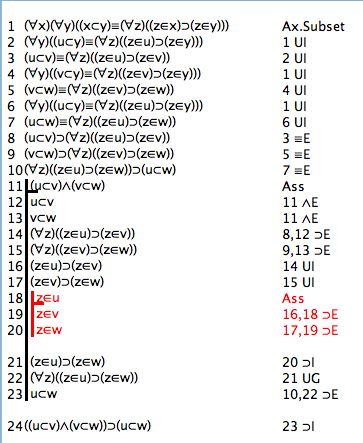# The Proof Widget

Logical System

2013

Some predicate logic proofs or derivations using Gentzen calculus. Try to prove them. Click on a line to select it. Select one or more lines, apply the appropriate rule off the Rules menu. (Click 'Derive It' off the Wizard Menu, if you want help).

Derive the following

(a) Fa ∴ (∀x)Fa
(b) (∀x) (Fx∧Gx) ∴ (∀x)Fx
(c) (∀x) (Fx∧Gx) ∴ (∀y)Fy
(d) ∴ (∀x)Fx≡(∀y)Fy
(e) (∀x) (Fx∧Gx) ∴ (∀y)Fy∧(∀z)Gz
(f) ∴ (∀x) (Fx∧Gx) ≡ (∀y)Fy∧(∀z)Gz
(g) ∴ (∀x) (Fx∧Gx) ≡ (∀x)Fx∧(∀x)Gx

#### Different systems

if you'd rather work with different logical symbols, you can do that

Derive the following

(a) Fa ∴ (x)Fa
(b) (x) (Fx.Gx) ∴ (x)Fx
(c) (x) (Fx.Gx) ∴ (y)Fy
(d) ∴ (x)Fx≡(y)Fy
(e) (x) (Fx.Gx) ∴ (y)Fy.(z)Gz
(f) ∴ (x) (Fx.Gx) ≡ (y)Fy.(z)Gz
(g) ∴ (x) (Fx.Gx) ≡ (x)Fx.(x)Gx

#### And there is set theory

There is the notion that whenever an element is a member of one set then it is also a member of a second set. When this occurs the first set is said to be a subset of the second, and this is denoted by the symbol '⊂' . There is an axiom covering this

(x⊂y) ≡(∀z)(zεx⊃zεy)     Axiom of Subsets

In English this says, 'x is a subset of y if, and only if, All z, if z is a member of x then z is also a member of y' .

[We certainly could have established this by means of a definition and the Axiom Schema of Abstraction, as explained earlier.]

And now we (ie you) can prove some theorems

1. ∴ ((u⊂v)∧ (v⊂w))⊃ (u⊂w)      /*The transitivity of the subset relation. Help Video on how to do this.*/
2. ∴ ((u⊂v)∧ (v⊂u))⊃ (u=v)      /*If two sets are subsets of each other, they are equal to each other.*/
3. ∴ (u=v)⊃ ((u⊂v)∧ (y⊂v))      /*If two sets are equal to each other, they are subsets of each other.*/

You will find the Axioms for Set Theory under the Advanced Menu, and all 3 of these proofs involve Universal Genalization and Instantiation steps to get the variables with the names you want.

Here is a proof of theorem 1.#### Learning even more from mathematicians on making our proofs simpler

There is an awkwardness in our formal apparatus. It is to do with the facts that often we want to use xs and ys in our formulas, and that our axioms also use xs and ys; this sometimes forces us into 'changing bound variables' and all sorts of purely formal manipulations. These formal steps are nothing to do with set theory (and, in fact, are a waste of time as far as learning set theory goes). [The difficulties here are the reason why us, vs and ws were used in Theorems 1-3.]

When working mathematicians look at, say

(x⊂y) ≡(∀z)(zεx⊃zεy)     Axiom of Subsets

they know that the xs and ys can have, or be instantiated to, any values. They see the xs and ys as being patterns and then those patterns can be matched to any values. We can do the same. We can take the Axiom of Subsets (and the Axiom of Extensionality) to be rewrite rules. So here are the rules.

(x=y)::(∀z)(zεx≡zεy))     Rewrite Extensionality

(x⊂y)::(∀z)(zεx⊃zεy)     Rewrite Subsets

[You might want to revise earlier material on rewriting. Easy Deriver Rewrites. Rewrite Rules are found on the Advanced Menu under Rewrites.]

Here is the same theorem using rewritesAnd here is a Help Video showing that.

#### To continue with Set Theory

[There is a point of notation. When one set is a subset of another, often it is possible for the first to actually equal the second. Some writers acknowledge this by using the symbol '⊆' so that a⊆b is read 'a is a subset of b or is equal to it; then '⊂' as in a⊂b is 'a is a subset of b but is not equal to it'. If a set is a subset of another but is not equal to it, the first is said to be a proper subset of the second. We are not using any of these conventions here. For us, a⊂b is 'a is a subset of b or is equal to it' and a⊂b∧~(a=b) says that 'a is a proper subset of b' (for it is a subset and it is not equal).]

There is the empty or null set. This is a set that nothing is a member of, it has the symbol '∅' .

(∀x)(x∉∅)    Axiom of Empty Set

In English this says, 'Nothing is a member of the empty set'. [There is the alternative notation of '{}' for the empty set-- we are not really using that here. The software will read {} but it does not know that (∀x)(x∉{}). So, if you want to use that convention in your proofs, you will need an extra premise that ∅={} .]

There is a symbolic convention in use here 'not a member of' has the symbol '∉' so, for example, ~(xε∅) can be abbreviated as (x∉∅) .

There is the universe or universal set. This is a set that everything is a member of, it has the symbol 'Ü'  (type option-U upper-case-U).

(∀x)(xεÜ)    Axiom of Universe Set

In English this says, 'Everything is a member of the Universal set' .

More theorems

4. ∴ ∅⊂x      /*The Empty set is a subset of every set. */
5. ∴ x⊂Ü      /*Every set is a subset of the Universe set.*/